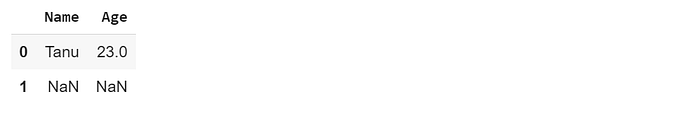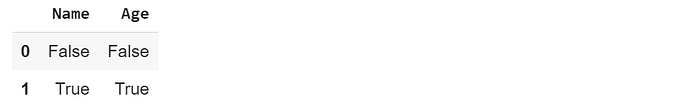# Pandas DataFrame: A Complete Introduction for BeginnersIn this article, you'll learn the basics of Pandas DataFrame from scratch.

Before getting started let me introduce you about

Pandas, Pandas is a python library which provides high-performance, easy-to-use data structures such as a series, Data Frame and Panel for data analysis tools for Python programming language. Moreover, Pandas Data Frame consists of main components, the data, rows, and columns. To use the pandas library and its data structures, all you have to do it to install it and import it. See the documentation of the Pandas library for a better understanding and installing guidance.

Basic operations that can be applied on a pandas Data Frame are as shown below:

1. Creating a Data Frame.
2. Performing operations on Rows and Columns.
4. Working with missing data.
5. Renaming the Columns or Indices of a DataFrame.

## 1. Creating a Data Frame.

The pandas data frame can be created by loading the data from the external, existing storage like a database, SQL or CSV files. But the pandas Data Frame can also be created from the lists, dictionary, etc. One of the ways to create a pandas data frame is shown below:

``````# import the pandas library
import pandas as pd
# Dictionary of key pair values called data
data = {'Name':['Ashika', 'Tanu', 'Ashwin', 'Mohit', 'Sourabh'],
'Age': [24, 23, 22, 19, 10]}
data

{'Age': [24, 23, 22, 19, 10],  'Name': ['Ashika', 'Tanu', 'Ashwin', 'Mohit', 'Sourabh']}
# Calling the pandas data frame method by passing the dictionary (data) as a parameter
df = pd.DataFrame(data)
df
``````## 2. Performing operations on Rows and Columns.

Data Frame is a two-dimensional data structure, data is stored in rows and columns. Below we can perform some operations on Rows and Columns.*Selecting a Column: *

In order to select a particular column, all we can do is just call the name of the column inside the data frame.

``````# import the pandas library
import pandas as pd
# Dictionary of key pair values called data
data = {'Name':['Ashika', 'Tanu', 'Ashwin', 'Mohit', 'Sourabh'],
'Age': [24, 23, 22, 19, 10]}
data

{'Age': [24, 23, 22, 19, 10],  'Name': ['Ashika', 'Tanu', 'Ashwin', 'Mohit', 'Sourabh']}
# Calling the pandas data frame method by passing the dictionary (data) as a parameter
df = pd.DataFrame(data)
# Selecting column
df[['Name']]
``````*Selecting a Row: *

Pandas Data Frame provides a method called “loc” which is used to retrieve rows from the data frame. Also, rows can also be selected by using the “iloc” as a function.

``````# Calling the pandas data frame method by passing the dictionary (data) as a parameter
df = pd.DataFrame(data)
# Selecting a row
row = df.loc
row

Name    Tanu
Age       23
Name: 1, dtype: object
``````

To select a particular column, all we can do is just call the name of the column inside the data frame. As seen above to work with the “loc” method you have to pass the index of the data frame as a parameter. The loc method accepts only integers as a parameter. So in the above example, I wanted to access “Tanu” row, so I passed the index as 1 as a parameter. Now there's a quick assignment for you guys, use the “iloc” method and tell me the result.

## 3. Data Selection, addition, deletion.

You can treat a DataFrame semantically like a dictionary of like-indexed Series objects. Getting, setting, and deleting columns works with the same syntax as the analogous dictionary operations:

``````# import the pandas library
import pandas as pd
# Dictionary of key pair values called data
data = {'Name':['Ashika', 'Tanu', 'Ashwin', 'Mohit', 'Sourabh'],
'Age': [24, 23, 22, 19, 10]}

# Calling the pandas data frame method by passing the dictionary (data) as a parameter
df = pd.DataFrame(data)
# Selecting the data from the column
df['Age']
0    24
1    23
2    22
3    19
4    10
Name: Age, dtype: int64
``````

Columns can be deleted like with a dictionary just use the del operation.

``````del df[‘Age’]
df
``````Data can be added by using the insert function. The insert function is available to insert at a particular location in the columns:

``````df.insert(1, ‘name’, df[‘Name’])
df
``````## 4. Working with missing data.

Missing data occur a lot of times when we are accessing big data sets. It occurs often like NaN (Not a number). In order to fill those values, we can use “isnull()” method. This method checks whether a null value is present in a data frame or not.Checking for the missing values.

``````# importing both pandas and numpy libraries
import pandas as pd
import numpy as np

# Dictionary of key pair values called data
data ={‘First name’:[‘Tanu’, np.nan],
‘Age’: [23, np.nan]}
df = pd.DataFrame(data)
df
````````````# using the isnull() function
df.isnull()
``````The isnull () returns false if the null is not present and true for null values. Now we have found the missing values, the next task is to fill those values with 0 this can be done as shown below:

``````df.fillna(0)
``````## 5. Renaming the Columns or Indices of a DataFrame.

To give the columns or the index values of your data frame a different value, it’s best to use the .rename() method. Purposefully I have changed the column name to give a better insight.

``````# import the pandas library
import pandas as pd
# Dictionary of key pair values called data
data = {‘NAMe’:[‘Ashika’, ‘Tanu’, ‘Ashwin’, ‘Mohit’, ‘Sourabh’],
‘AGe’: [24, 23, 22, 19, 10]}

# Calling the pandas data frame method by passing the dictionary (data) as a parameter
df = pd.DataFrame(data)
df
````````````newcols = {
‘NAMe’: ‘Name’,
‘AGe’: ‘Age’
}
# Use `rename()` to rename your columns
df.rename(columns=newcols, inplace=True)
df
````````````# The values of new index
newindex = {
0: ‘a’,
1: ‘b’,
2: ‘c’,
3: ‘d’,
4: ‘e’
}
df.rename(index=newindex)
``````Hence above are the very important techniques or methods of pandas data frame in Python. If you guys have some doubts in the code, the comment section is all yours.Thank you.

## Python Pandas Tutorial - Data Analysis with Python Pandas

Python Pandas Tutorial will help you get started with Python Pandas Library for various applications including Data analysis. Introduction to Pandas. DataFrames and Series. How To View Data? Selecting Data. Handling Missing Data. Pandas Operations. Merge, Group, Reshape Data. Time Series And Categoricals. Plotting Using Pandas

## Python For Data Analysis | Build a Data Analysis Library from Scratch | Learn Python in 2019

Python For Data Analysis - Build a Data Analysis Library from Scratch - Learn Python in 2019

## Data Science Course in Dallas

Become a data analysis expert using the R programming language in this [data science](https://360digitmg.com/usa/data-science-using-python-and-r-programming-in-dallas "data science") certification training in Dallas, TX. You will master data...